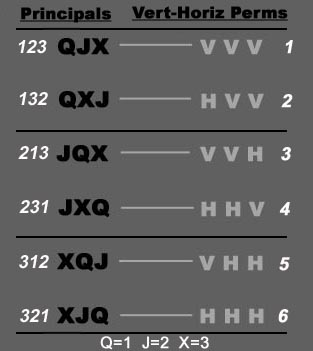Each Toperm is the product of combining a principal perm with a polar perm. The six principal perms are shown numerically with their respective arrangements on the left (e.g. 312=XQJ) and are numbered consecutively 1-6 along the right.The eight polar perms are sexed by yang or yin, where odd numbers in the Toperm are yang (1)and even numbers are yin(2). Take, for instance, the Toperm 614 =XQJ (3 1 2) with Yin-Yang-Yin applied to the principals (2 1 2). The polar trigrams are erected from the bottom line upward, so in this example we see:The combination of both the principal & polar components of an exact face are called a Paraperm. In this instance (#21, Averse E Vex) the paraperm is 5-6; expanded to its form 312-212 . There are 6 principal perms x 8 polar perms = 48 total paraperms. Each paraperm corresponds to an exact face as illustrated in the complete table below.As always, the table should be worked through & verified with your own set of tablocks.

NEXT